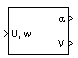# Incidence & Airspeed

Calculate incidence and airspeed

• Library:
• Aerospace Blockset / Flight Parameters

•## Description

The Incidence & Airspeed block supports the 3DoF equations of motion model by calculating the angle between the velocity vector and the body, and also the total airspeed from the velocity components in the body-fixed coordinate frame.

`$\begin{array}{l}\alpha =\text{atan}\left(\frac{w}{u}\right)\\ V=\sqrt{{u}^{2}+{w}^{2}}\end{array}$`

## Ports

### Input

expand all

Velocity of the body, specified as a two-element vector, resolved into the body-fixed coordinate frame.

Data Types: `double`

### Output

expand all

Incidence angle, returned as a scalar, in radians.

Data Types: `double`

Airspeed of the body, returned as a scalar.

Data Types: `double`

## Extended Capabilities

### C/C++ Code GenerationGenerate C and C++ code using Simulink® Coder™.